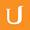## Practice 3 - Visualizing Algebra

• 0:00 - 0:04
Did you get the closed interval from negative 1 to 1? If so, you should be very
• 0:04 - 0:08
proud of yourself. I know I am. Let's see how we got that solution. Remember
• 0:08 - 0:12
when you're working with a compound inequality that's written in this form, we
• 0:12 - 0:16
can perform operations as long as we do the same operation, to all three
• 0:17 - 0:21
portions of the quation. The first thing I want to do is to get rid of this
• 0:21 - 0:26
fraction by multiplying through by the least common denominator, and the least
• 0:26 - 0:31
common denominator is 2. When I multiply 2 by negative 3, I get negative 6. When
• 0:31 - 0:36
I multiply 2 by 5 x minus 1 over 2, I'm simply left with 5 x minus 1, and when I
• 0:36 - 0:40
multiply 2 times 2, I get 4. Next I'm going to add 1 to each part of the
• 0:40 - 0:46
equation so I can isolate the variable in the middle. When I do that, negative 6
• 0:46 - 0:51
plus 1 is negative 5, and 4 plus 1 is positive 5. Now I can divide through by 5.
• 0:51 - 0:56
Negative 5 divided by 5 is negative 1, less than or equal to x, less than or
• 0:56 - 1:01
equal to 5 over 5 is positive 1. Let's draw that on the number line. This
• 1:01 - 1:06
portion of the inequality says that negative 1 is less than or equal to x, which
• 1:06 - 1:11
is the same as x is greater than or equal to negative 1. So, that means we're
• 1:11 - 1:14
going to be going in that direction.
• 1:14 - 1:18
This portion of the equation says x is less than or equal to positive 1, so we
• 1:18 - 1:23
use a square bracket and the answer's going here. So we have the interval from
• 1:23 - 1:26
negative 1 to 1 using the square brackets.
Title:
Practice 3 - Visualizing Algebra
Video Language:
English
Team:Udacity
Project:
MA006 - Visualizing Algebra
Duration:
01:27Udacity Robot edited English subtitles for Practice 3 - Visualizing AlgebraCogi-Admin added a translation

# English subtitles

## Revisions Compare revisions

• API
Udacity Robot